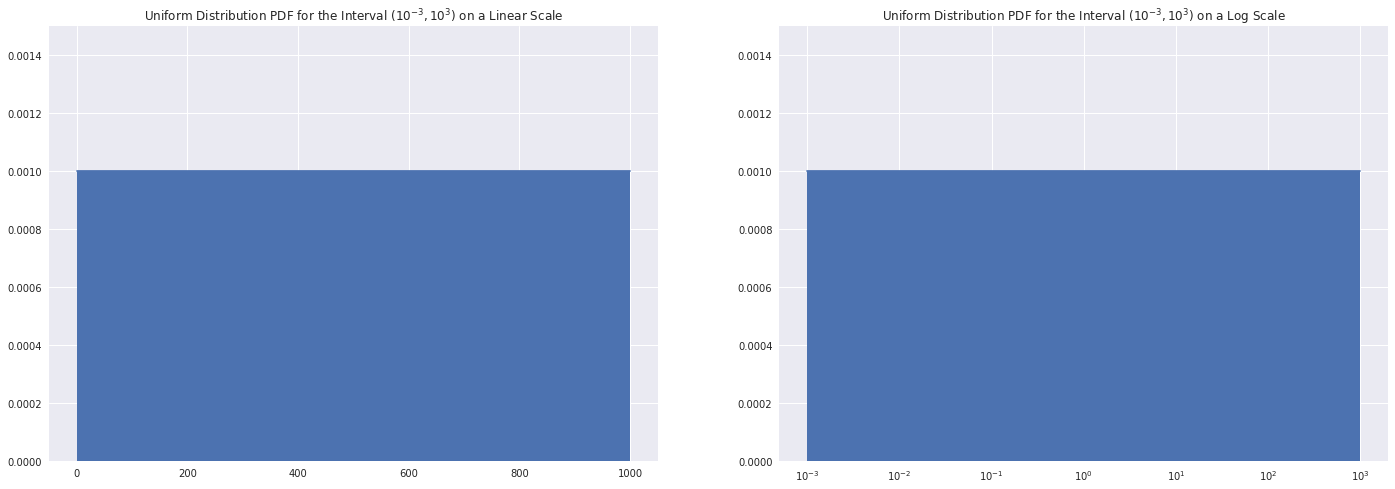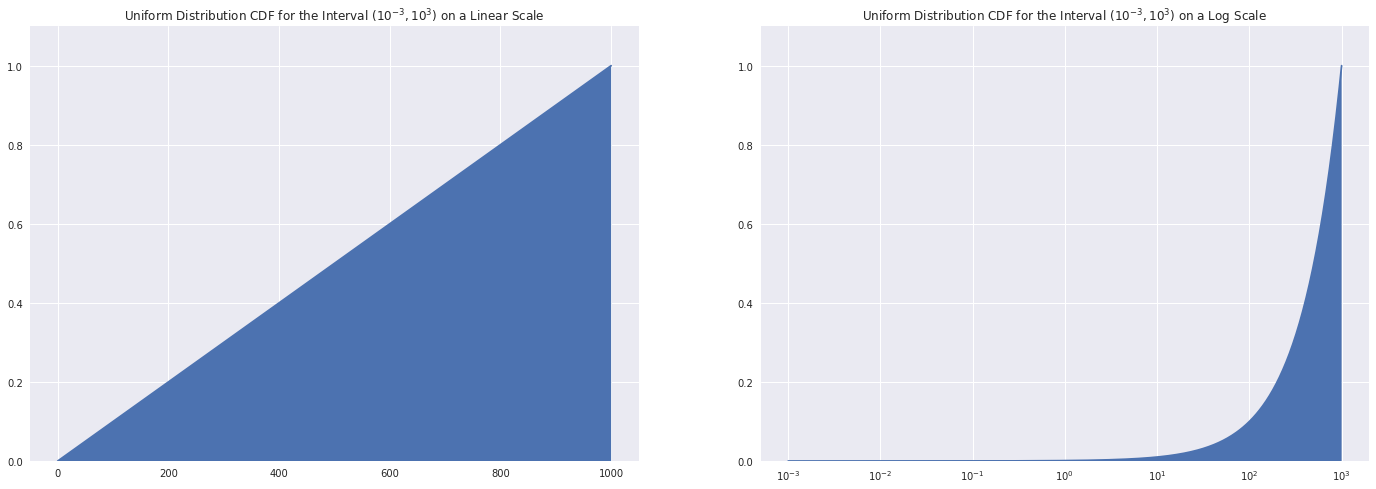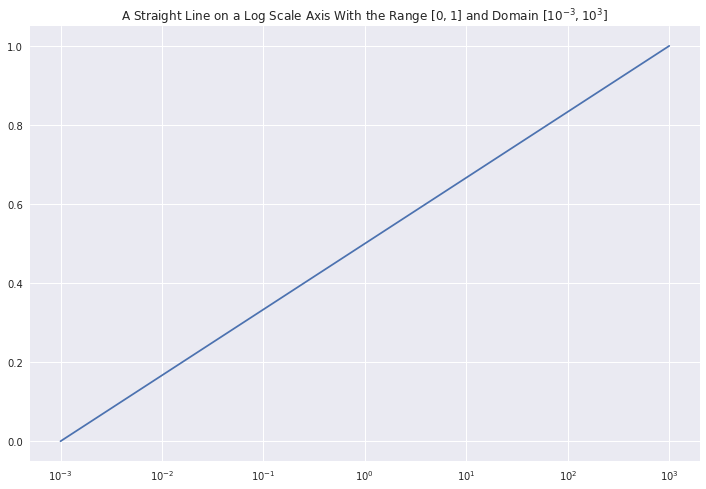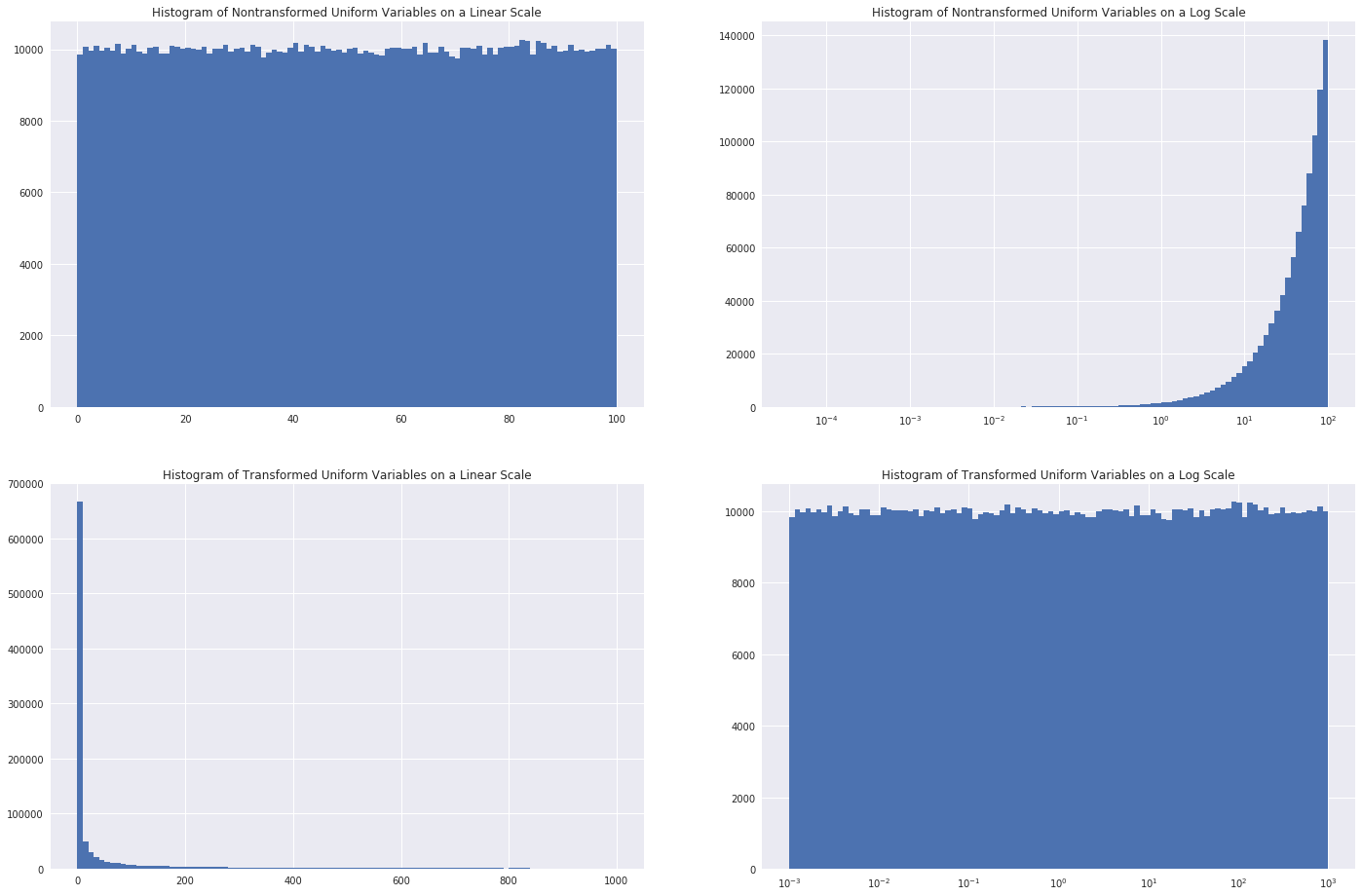Tim's Blog

## Distributions for Randomized SearchCV

06 Oct 2018

A central part of data science is hyperparameter optimization, which can often be a difficult challenge to overcome. The process of hyperparameter optimization can be computationally expensive and prone to both underfitting and overfitting if not carried out well. However, one of the best methods that exist for hyperparameter optimization is a randomized search with cross-validation, like sklearn.model_selection.RandomizedSearchCV. This method is not nearly as computationally expensive as a grid search, and also provides a balance between overfitting and underfitting. The only issue in using randomized search is selecting a distribution that parameter values are to be drawn from. In this blog post, I will talk about one of the biggest issues with randomized search: sampling probability distributions on non-linear scales. Below, I propose a function to map random samples of a probability distribution from a linear scale to a logarithmic scale.

A central part of data science is hyperparameter optimization, which can often be a difficult challenge to overcome. The process of hyperparameter optimization can be computationally expensive and prone to both underfitting and overfitting if not carried out well. However, one of the best methods that exist for hyperparameter optimization is a randomized search with cross-validation, like sklearn.model_selection.RandomizedSearchCV. This method is not nearly as computationally expensive as a grid search, and also provides a balance between overfitting and underfitting. The only issue in using randomized search is selecting a distribution that parameter values are to be drawn from. In this blog post, I will talk about one of the biggest issues with randomized search: sampling probability distributions on non-linear scales. Below, I propose a function to map random samples of a probability distribution from a linear scale to a logarithmic scale.

import numpy as np
import pandas as pd
from scipy import stats
from sklearn.model_selection import RandomizedSearchCV
from sklearn.linear_model import LogisticRegression
import matplotlib.pyplot as plt
import seaborn as sns
import time
import warnings

warnings.filterwarnings('ignore')
sns.set(style = "darkgrid")

xsize = 12.0
ysize = 8.0


When tuning hyperparameters, we often care about the order of magnitude of a parameter more than its actual value. This is because trying to hone in on an exact number for a hyperparameter can lead to overfitting. Furthermore, most model hyperparameters result in nonlinear effects in the model itself, so increasing a hyperparameter by an order of magnitude will not necessecarily change the model’s accuracy by an order of magnitude. Therefore, it is quite natural to think of hyperparameters in terms of a log scale.

When using a randomized search method, we need to provide it with a probability distribution to sample hyperparameters from. Unfortunately, most distributions are linearly scaled not log scaled. By nature, every order of magnitude is a constant multiple of the previous order; for example, $10^n = 10\cdot 10^{n-1}$. This is problematic because it causes random samples of probability distributions to favor the largest order of magnitude. To illustrate this, let $p_{min} = 10^{-3}$ and $p_{max} = 10^3$, and consider the uniform probability distribution over that domain: $U\left(10^{-3}, 10^3\right)$.

p_max = 1e3
p_min = 1e-3
linear_sample_points = np.linspace(p_min, p_max, 500)
geometric_sample_points = np.geomspace(p_min, p_max, 500)

fig, (ax1, ax2) = plt.subplots(ncols = 2)
fig.set_size_inches(2.0*xsize, ysize)

ax1.plot(linear_sample_points, stats.uniform.pdf(linear_sample_points, loc = p_min, scale = p_max))
ax1.fill_between(linear_sample_points, stats.uniform.pdf(linear_sample_points, loc = p_min, scale = p_max), np.zeros(len(linear_sample_points)))
ax1.set_title("Uniform Distribution PDF for the Interval "+r"$\left(10^{-3}, 10^{3}\right)$"+" on a Linear Scale")
ax1.set_ylim((0.0, 0.0015))

ax2.semilogx(geometric_sample_points, stats.uniform.pdf(geometric_sample_points, loc = p_min, scale = p_max))
ax2.fill_between(geometric_sample_points, stats.uniform.pdf(geometric_sample_points, loc = p_min, scale = p_max), np.zeros(len(geometric_sample_points)))
ax2.set_title("Uniform Distribution PDF for the Interval "+r"$\left(10^{-3}, 10^{3}\right)$"+" on a Log Scale")
ax2.set_ylim((0.0, 0.0015))

plt.show()The graph on the left shows the PDF of the distribution $U$ on a linear scale, while the graph on the right shows the PDF of the distribution $U$ on a logarithmic scale. At a glance, the PDF appears to be the same on both scales, which which is misleading as the uniform distribution is not the same both on a linear and logarithmic scale, even if their PDFs look similar.

fig, (ax1, ax2) = plt.subplots(ncols = 2)
fig.set_size_inches(2.0*xsize, ysize)

ax1.plot(linear_sample_points, stats.uniform.cdf(linear_sample_points, loc = p_min, scale = p_max))
ax1.fill_between(linear_sample_points, stats.uniform.cdf(linear_sample_points, loc = p_min, scale = p_max), np.zeros(len(linear_sample_points)))
ax1.set_title("Uniform Distribution CDF for the Interval "+r"$\left(10^{-3}, 10^{3}\right)$"+" on a Linear Scale")
ax1.set_ylim((0.0, 1.1))

ax2.semilogx(geometric_sample_points, stats.uniform.cdf(geometric_sample_points, loc = p_min, scale = p_max))
ax2.fill_between(geometric_sample_points, stats.uniform.cdf(geometric_sample_points, loc = p_min, scale = p_max), np.zeros(len(geometric_sample_points)))
ax2.set_title("Uniform Distribution CDF for the Interval "+r"$\left(10^{-3}, 10^{3}\right)$"+" on a Log Scale")
ax2.set_ylim((0.0, 1.1))

plt.show()Above on the left is the CDF of the distribution $U$ on a linear scale and on the right is the CDF of the same distribution $U$ on a logarithmic scale. Unlike the PDF from before the CDF is clearly not the same on both a linear and logarithmic scale. This is because the PDF of the uniform distribution on $\left[\alpha,\beta\right]$ is given by,

which is not dependent on $x$. However, the CDF of the uniform distribution is just the integral of $f(x)$ with respect to the measure. On a linear scale this measure is $\mathbb{d}x$, but on a logarithmic scale this measure is $\mathbb{d}\log\left(x\right)$, such that,

Furthermore, if you visually inspect the graph on the right above it appears that the value of the CDF of the uniform distribution at $x=10^2$ is approximately $0.1$ whereas the value of the CDF at $x=10^3$ is $1$, which demonstrates the above property about probability distributions favoring larger orders of magnitude.

Therefore, we desire a function that maps the linear interval $\left[0,1\right]$ to the logarthimic interval $\left[a,b\right]$. We start the process of deriving $F$ by recalling that a straight line on a logarthmic measure with the points $\left(a, 0\right)$ and $\left(b,1\right)$ is given by,

which is plotted below with $a=p_{min}$ and $b=p_{max}$.

def logaxisline(x, a, b):
return (np.log10(x) - np.log10(a))/(np.log10(b) - np.log10(a))

fig, ax = plt.subplots()
fig.set_size_inches(xsize, ysize)

ax.set_title("A Straight Line on a Log Scale Axis With the Range "+r"$\left[0,1\right]$"+" and Domain "+r"$\left[10^{-3}, 10^{3}\right]$")
ax.semilogx(np.geomspace(p_min, p_max, 500), logaxisline(np.geomspace(p_min, p_max, 500), p_min, p_max))

plt.show()Then to find the desired function $F\left(x\right) : \left[0,1\right] \rightarrow \left[a,b\right]$, we solve for the inverse of the straight line above,

Which yields,

This actually turns out to be a rather nice formula. To demonstrate how this function behaves, consider the standard uniform distribution, $U\left(0,1\right)$, and $a=p_{min}$ and $b=p_{max}$.

def F(x, a, b):
return np.power(a, 1.0 - x)*np.power(b, x)

s = int(1e6)
uniform_vars = stats.uniform.rvs(size = s)
transformed_uniform_vars = F(uniform_vars, p_min, p_max)

fig, ((ax1, ax2), (ax3, ax4)) = plt.subplots(nrows = 2, ncols = 2)
fig.set_size_inches(2.0*xsize, 2.0*ysize)

_, bins = np.histogram(uniform_vars, bins = 100)
logbins = np.logspace(np.log10(bins), np.log10(bins[-1]), len(bins))
ax1.hist(uniform_vars, bins = bins)
ax1.set_title("Histogram of Nontransformed Uniform Variables on a Linear Scale")

ax2.hist(uniform_vars, bins = logbins)
ax2.set_xscale("log")
ax2.set_title("Histogram of Nontransformed Uniform Variables on a Log Scale")

_, bins = np.histogram(transformed_uniform_vars, bins = 100)
logbins = np.logspace(np.log10(bins), np.log10(bins[-1]), len(bins))
ax3.hist(transformed_uniform_vars, bins = bins)
ax3.set_title("Histogram of Transformed Uniform Variables on a Linear Scale")

ax4.hist(transformed_uniform_vars, bins = logbins)
ax4.set_xscale("log")
ax4.set_title("Histogram of Transformed Uniform Variables on a Log Scale")

plt.show()Above in the top left is a histogram of random samples from $U\left(0,1\right)$ on a linear scale, which qualitatively appears to be roughly uniform as expected. In the top right is a histogram of random samples from $U\left(0,1\right)$ on a logarithmic scale, which shows the same behavior from before and further demonstrates why a linearly scaled uniform distribution should not be used for tuning hyperparameters. In the bottom left is a histogram of the same samples after being transformed by the function $F\left(x\right)$ on a linear scale, which qualitatively appears to be a kind of inverse for the top right graph. Finally, in the bottom right is a histogram of the same random samples after being transformed by the function $F\left(x\right)$ on a logarithmic scale, which demonstrates the power of the transform.

However, there are many other continuous bounded distributions that would be nice to have in our toolbox for randomized parameter estimation. In this case we would like a function $F : \left[\alpha, \beta\right] \rightarrow \left[a, b\right]$, where $\left[\alpha,\beta\right]$ is the domain of the linearly scaled distribution and $\left[a,b\right]$ is the domain of the logarithmically scaled distribution. This can be found by solving the expression below for the inverse, which is just a slightly modified version of the straight line on a logarithmic scale equation from earlier.

This yields,

To demonstrate this more generalized version of $F\left(x\right)$ let’s consider the example of a uniform distribution, $U\left(0, 100\right)$, so that $\alpha=0$, $\beta=100$, $a=p_{min}$, and $b=p_{max}$.

def F2(x, a, b, alpha, beta):
return np.exp((np.log(a)*(x - beta) + np.log(b)*(alpha - x))/(alpha - beta))

s = int(1e6)
uniform_vars2 = stats.uniform.rvs(loc = 0.0, scale = 100.0, size = s)
transformed_uniform_vars2 = F2(uniform_vars2, p_min, p_max, 0.0, 100.0)

fig, ((ax1, ax2), (ax3, ax4)) = plt.subplots(nrows = 2, ncols = 2)
fig.set_size_inches(2.0*xsize, 2.0*ysize)

_, bins = np.histogram(uniform_vars2, bins = 100)
logbins = np.logspace(np.log10(bins), np.log10(bins[-1]), len(bins))
ax1.hist(uniform_vars2, bins = bins)
ax1.set_title("Histogram of Nontransformed Uniform Variables on a Linear Scale")

ax2.hist(uniform_vars2, bins = logbins)
ax2.set_xscale("log")
ax2.set_title("Histogram of Nontransformed Uniform Variables on a Log Scale")

_, bins = np.histogram(transformed_uniform_vars2, bins = 100)
logbins = np.logspace(np.log10(bins), np.log10(bins[-1]), len(bins))
ax3.hist(transformed_uniform_vars2, bins = bins)
ax3.set_title("Histogram of Transformed Uniform Variables on a Linear Scale")

ax4.hist(transformed_uniform_vars2, bins = logbins)
ax4.set_xscale("log")
ax4.set_title("Histogram of Transformed Uniform Variables on a Log Scale")

plt.show()The graphs above each represent the same thing as in the less general case of $F\left(x\right)$ but demonstrate that the more general version of $F\left(x\right)$ preserves all the same scaling properties. Unfortunately, $F\left(x\right)$ does come with a set of restrictions. First, $b>a>0$ otherwise $F\left(x\right)$ is undefined. Second, $\infty>\beta>\alpha\geq 0$, which means $\alpha$ must be greater than or equal to zero and $\beta$ must be bounded as well. The second restriction eliminates many continuous distributions, such as a normal or lognormal distribution, from being used because many continuous distributions have the domain of either $\left(-\infty,\infty\right)$ or $\left[0,\infty\right)$. However, the simplest fix for this would be to truncate an unbounded or semibounded continuous distribution to some appropriate $\left[\alpha,\beta\right]$ and then find the normalization constant for that truncated distribution.

Finally, I’ll use this function $F\left(x\right)$ with sklearn.model_selection.RandomizedSearchCV to demonstrate the use of this function through a data science application. First, I’ll define a class that will take a probability distribution and has the rvs method that RandomizedSearchCV expects.

class LogScaledDistribution(object):
def __init__(self, dist, a, b, alpha = 0.0, beta = 1.0, dist_args = None):
if dist_args == None:
self.dist = dist()
else:
self.dist = dist(**dist_args)

self.a = a
self.b = b
self.alpha = alpha
self.beta = beta

def _F(self, x):
return np.exp((np.log(self.a)*(x - self.beta) + np.log(self.b)*(self.alpha - x))/(self.alpha - self.beta))

def rvs(self, size = None, random_state = None):
if type(s) == type(None):
return self._F(self.dist.rvs(random_state = random_state))
else:
return self._F(self.dist.rvs(size = size, random_state = random_state))


Finally, I’ll use the LogScaledDistribution to tune a logistic regression using contrived data to demonstrate that the transformation and wrapper class around it actually work and are compatible with sklearn’s API.

X = np.random.randn(100, 5)
y = np.sum(X, axis = 1)
y[y > 0.5] = 1.0
y[y <= 0.5] = 0.0

logistic_regression = LogisticRegression(dual = False, tol = 0.0001, fit_intercept = True, class_weight = None,
random_state = None, solver = "liblinear", max_iter = 100,
multi_class = "ovr", verbose = 0, warm_start = False, n_jobs = 1)

params = {
"penalty": ["l2", "l1"],
"C": LogScaledDistribution(stats.uniform, 1e-1, 1e1),
"intercept_scaling": LogScaledDistribution(stats.uniform, 1e-1, 1e1)
}

clf = RandomizedSearchCV(logistic_regression, params, n_iter = 10, scoring = None, fit_params = None,
n_jobs = 1, iid = True, refit = True, cv = 10, verbose = 0, pre_dispatch = "2*n_jobs",
random_state = None, error_score = "raise", return_train_score = "warn")
clf.fit(X, y)
print(clf.best_params_)
print(clf.score(X, y))

{'C': 9.638368580773934, 'intercept_scaling': 5.5132833456900405, 'penalty': 'l1'}
1.0


In conclusion, I’ve derived a function that can take a random sample from a linearly scaled probability distribution and map it to a random sample from a logarithmically scaled probability distribution, which is useful for randomized search hyperparameter optimization. Also, the class that I made above will likely find quite a bit of use in my future data science projects. A future area of research would be finding a way to map an unbounded or semibounded probability distribution to a logarithmic scale without truncating or how to map linearly scaled discrete probability distributions to a logarithmic scale.

Finally, I’d like to give a special thanks to Robert van der Drift for help with editing this blog post.

A jupyter notebook version of this blog post can be found here.

Tweet me @https://twitter.com/TimTimWillard if you like this post.